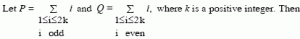GeeksforGeeks App
Open AppBrowser
Continue

# GATE | GATE CS 2008 | Question 24(A) P = Q – k
(B) P = Q + k
(C) P = Q
(D) P = Q +2 k

Explanation:

```P is sum of odd integers from 1 to 2k

Q is sum of even integers from 1 to 2k

Let k = 5

P is sum of odd integers from 1 to 10
P = 1 + 3 + 5 + 7 + 9

Q is sum of even integers from 1 to 10
Q = 2 + 4 + 6 + 8 + 10

In general, Q can be written as
Q = (1 + 3 + 5 + 9..... ) + (1 + 1 + .....)
= P + k
```
My Personal Notes arrow_drop_up